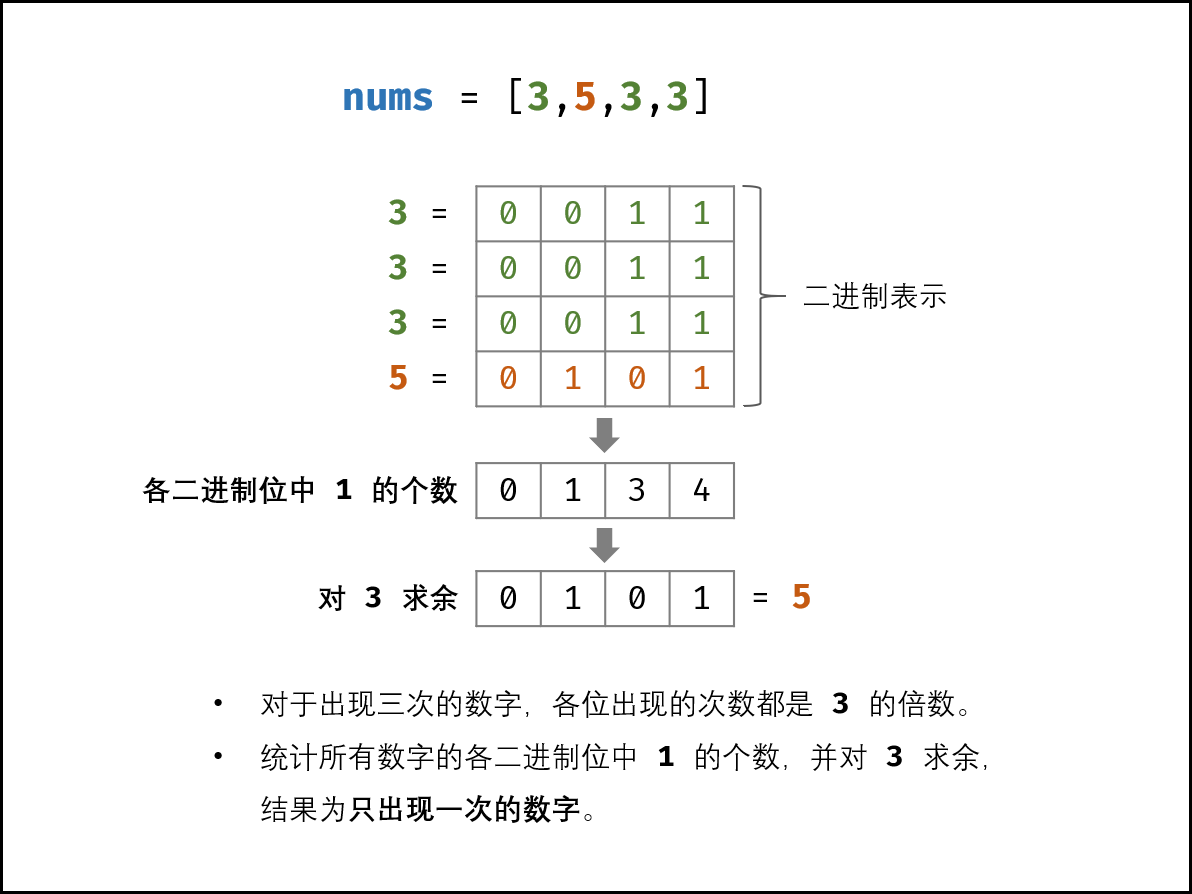# 剑指 Offer（专项突击版）第3|4题

## 前言

• 现在前端要求变高了,找工作的时可能会碰上算法题,每天刷几道算法题做足准备,今天是《剑指 Offer（专项突击版）》第3|4题。

## 剑指 Offer II 003. 前 n 个数字二进制中 1 的个数

``````示例 1:

● 0 <= n <= 105
``````

### 方法一：位运算

``````/**
* @param {number} n
* @return {number[]}
*/
var countBits = function(n) {
let result = new Array(n + 1).fill(0);;
for (let i = 0; i<=n; i++) {
let j = i;
while(j !== 0) {
result[i]++;
j = j & (j -1);
}
}
return result;
};
``````

• 时间复杂度：O(nlog⁡n)。需要对从 0 到 n 的每个整数使用计算「一比特数」，对于每个整数计算「一比特数」的时间都不会超过 O(log⁡n)。

• 空间复杂度：O(1)。除了返回的数组以外，空间复杂度为常数。

### 方法二：动态规划

``````/**
* @param {number} n
* @return {number[]}
*/
var countBits = function(n) {
let res = [];
res = 0
for(let i = 1;i <=n;i++) {
//整数 i 的二进制形式中1的个数比 i &(i - 1)的二进制形式中1的个数多1
res[i] = res[i & (i - 1)] + 1;
}
return res
};
``````

• 时间复杂度：O(n)。对于每个整数，只需要 O(1) 的时间计算「一比特数」。
• 空间复杂度：O(1)。除了返回的数组以外，空间复杂度为常数。

## 剑指 Offer II 004. 只出现一次的数字

``````示例 1：

● 1 <= nums.length <= 3 * 104
● -231 <= nums[i] <= 231 - 1
● nums 中，除某个元素仅出现 一次 外，其余每个元素都恰出现 三次
``````

### 方法一：位运算``````/**
* @param {number[]} nums
* @return {number}
*/
var singleNumber = function(nums) {
// 创建一个长度为32的数组，默认值为0
let arr = new Array(32).fill(0);
for (let i = 0; i<nums.length; i++) {
for (let j = 0; j<32; j++) {
// 整数i先被右移31-i位，原来从左起第i个数位右移之后位于最右边
// 与1做位与运算   也就是说只有1与1做位与运算才为1否则为0
arr[j] += (nums[i] >> (31 - j)) & 1;
}
}

// arr中每一位保存着nums中对应位中1的个数和
let res = 0;
for (let i = 0; i<32; i++) {
res = (res << 1) + (arr[i] %3);
}
return res;
};
``````

• 时间复杂度：O(n)
• 空间复杂度：O(1)

## so

• 结尾依旧：长风破浪会有时，直挂云帆济沧海！
• 在线整理的确实很好，对掘金的文章进行了一个汇总整理，在线刷题指南，拿走不谢，要学会站在别人的肩膀上提升自己点击这里–>  前端进阶指南## 传送门